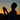WeAreOutMan

Dark

# JavaScript 作用域和闭包

March 24, 2014

JavaScript 中有两种作用域：函数作用域和全局作用域。

`function foo() {    var bar = 1;    {        var bar = 2;    }    return bar; // 2}`

`var bar = 1;function foo() {}alert(window.bar); // 1alert(window.foo); // "function foo() {}"`

### 作用域链

`var scope = 0, zero = "global-scope";(function(){    var scope = 1, one = "scope-1";    (function(){        var scope = 2, two = "scope-2";        (function(){            var scope = 3, three = "scope-3";            // scope-3 scope-2 scope-1 global-scope            console.log([three, two, one, zero].join(" "));            console.log(scope); // 3        })();        console.log(typeof three); // undefined        console.log(scope); // 2    })();    console.log(typeof two); // undefined    console.log(scope); // 1})();console.log(typeof one); // undefinedconsole.log(scope); // 0`

### 闭包

`function bind(func, target) {    return function() {        func.apply(target, arguments);    };}`

• 外部函数不能访问内嵌函数
• 外部函数也不能访问内嵌函数的参数和变量
• 而内嵌函数可以访问外部函数的参数和变量
• 换一个说法：内嵌函数包含了外部函数的作用域

`var scope = 0, zero = "global-scope";(function(){    var scope = 1, one = "scope-1";    (function(){        var scope = 2, two = "scope-2";        (function(){            var scope = 3, three = "scope-3";            // scope-3 scope-2 scope-1 global-scope            console.log([three, two, one, zero].join(" "));            console.log(scope); // 3        })();        console.log(typeof three); // undefined        console.log(scope); // 2    })();    console.log(typeof two); // undefined    console.log(scope); // 1})();console.log(typeof one); // undefinedconsole.log(scope); // 0`

• 每次外部函数的调用，内嵌函数都会被创建一次
• 在它被创建时，外部函数的作用域（包括任何本地变量、参数等上下文）， 会成为每个内嵌函数对象的内部状态的一部分，即使在外部函数执行完并退出后

`var i, list = [];for (i = 0; i < 2; i += 1) {    list.push(function(){        console.log(i);    });}list.forEach(function(func){  func();});`

`var i, list = [];for (i = 0; i < 2; i += 1) {    list.push((function(j){        return function(){            console.log(j);        };    })(i));}list.forEach(function(func){  func();});`Personal Blog by Shenwei Wang.
Writing code, raising kid, exploring the world.